# Ordinary differential equations, property C for

(diff) ← Older revision | Latest revision (diff) | Newer revision → (diff)

Let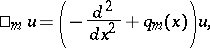and let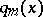be a real-valued function,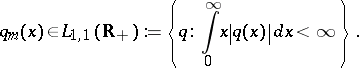Consider the problem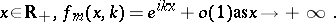This problem has a unique solution, which is called the Jost function.

Define also the solutions to the problem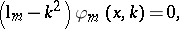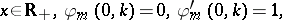and to the problem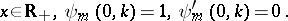Assume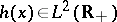and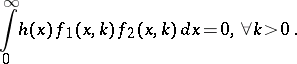(a1)

If (a1) implies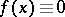, then one says that the pairhas property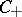.

Letbe an arbitrary fixed number, let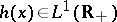and assume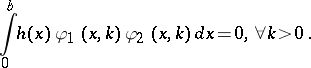(a2)

If (a2) implies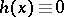, then one says that the pairhas property.

Similarly one defines property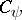.

It is proved in [a1] that the pair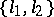has propertyif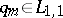,.

It is proved in [a2] that the pairhas properties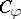and.

However, if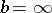, then, in general, propertyfails to hold for a pair. This means that there exist a function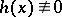,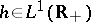, and two potentials, such that (a1) holds for all.

In [a2] many applications of properties,andto inverse problems are presented.

For instance, suppose that the-function, defined as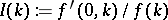, is known for all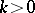,and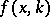is the Jost function corresponding to a potential.

The function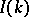is known as the impedance function [a4], and it can be measured in some problems of electromagnetic probing of the Earth. The inverse problem (IP) is: Givenfor all, can one recover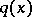uniquely?

This problem was solved in [a4], but in [a1] and [a2] a new approach to this and many other inverse problems is developed. This new approach is sketched below.

Suppose that there are two potentials,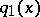and, which generate the same data. Subtract from the equation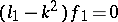the equation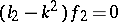, and denote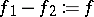,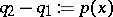, to get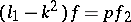. Multiply this equation by, integrate over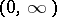and then by parts. The assumption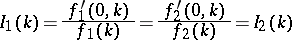implies,.

Using propertyone concludes, that is,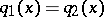. This is a typical scheme for proving uniqueness theorems using property.

How to Cite This Entry:
Ordinary differential equations, property C for. Encyclopedia of Mathematics. URL: http://encyclopediaofmath.org/index.php?title=Ordinary_differential_equations,_property_C_for&oldid=18905
This article was adapted from an original article by A.G. Ramm (originator), which appeared in Encyclopedia of Mathematics - ISBN 1402006098. See original article